# Siméon Denis Poisson facts for kids

Kids Encyclopedia Facts
Quick facts for kids
Siméon Poisson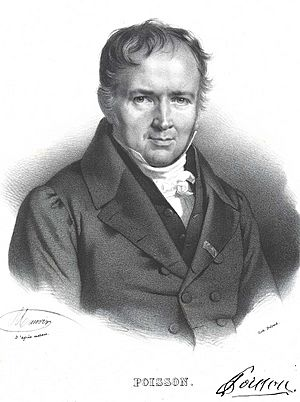Born 21 June 1781
Pithiviers, Kingdom of France
(present-day Loiret)
Died 25 April 1840 (aged 58)
Sceaux, Hauts-de-Seine, Kingdom of France
Alma mater École Polytechnique
Known for Poisson process
Poisson equation
Poisson kernel
Poisson distribution
Poisson bracket
Poisson algebra
Poisson regression
Poisson summation formula
Poisson's spot
Poisson's ratio
Poisson zeros
Conway–Maxwell–Poisson distribution
Euler–Poisson–Darboux equation
Scientific career
Fields Mathematics and physics
Institutions École Polytechnique
Bureau des Longitudes
Faculté des sciences de Paris [fr]
École de Saint-Cyr
Pierre-Simon Laplace
Doctoral students Michel Chasles
Joseph Liouville
Other notable students Nicolas Léonard Sadi Carnot
Peter Gustav Lejeune Dirichlet

Baron Siméon Denis Poisson FRS FRSE (French: [si.me.ɔ̃ də.ni pwa.sɔ̃]; 21 June 1781 – 25 April 1840) was a French mathematician and physicist who worked on statistics, complex analysis, partial differential equations, the calculus of variations, analytical mechanics, electricity and magnetism, thermodynamics, elasticity, and fluid mechanics. Moreover, he predicted the Poisson spot in his attempt to disprove the wave theory of Augustin-Jean Fresnel, which was later confirmed.

## Biography

Poisson was born in Pithiviers, Loiret district in France, the son of Siméon Poisson, an officer in the French army.

In 1798, he entered the École Polytechnique in Paris as first in his year, and immediately began to attract the notice of the professors of the school, who left him free to make his own decisions as to what he would study. In his final year of study, less than two years after his entry, he published two memoirs, one on Étienne Bézout's method of elimination, the other on the number of integrals of a finite difference equation and this was so impressive that he was allowed to graduate in 1800 without taking the final examination,. The latter of the memoirs was examined by Sylvestre-François Lacroix and Adrien-Marie Legendre, who recommended that it should be published in the Recueil des savants étrangers, an unprecedented honor for a youth of eighteen. This success at once procured entry for Poisson into scientific circles. Joseph Louis Lagrange, whose lectures on the theory of functions he attended at the École Polytechnique, recognized his talent early on, and became his friend. Meanwhile, Pierre-Simon Laplace, in whose footsteps Poisson followed, regarded him almost as his son. The rest of his career, until his death in Sceaux near Paris, was occupied by the composition and publication of his many works and in fulfilling the duties of the numerous educational positions to which he was successively appointed.

Immediately after finishing his studies at the École Polytechnique, he was appointed répétiteur (teaching assistant) there, a position which he had occupied as an amateur while still a pupil in the school; for his schoolmates had made a custom of visiting him in his room after an unusually difficult lecture to hear him repeat and explain it. He was made deputy professor (professeur suppléant) in 1802, and, in 1806 full professor succeeding Jean Baptiste Joseph Fourier, whom Napoleon had sent to Grenoble. In 1808 he became astronomer to the Bureau des Longitudes; and when the Faculté des sciences de Paris [fr] was instituted in 1809 he was appointed a professor of rational mechanics (professeur de mécanique rationelle). He went on to become a member of the Institute in 1812, examiner at the military school (École Militaire) at Saint-Cyr in 1815, graduation examiner at the École Polytechnique in 1816, councillor of the university in 1820, and geometer to the Bureau des Longitudes succeeding Pierre-Simon Laplace in 1827.

In 1817, he married Nancy de Bardi and with her, he had four children. His father, whose early experiences had led him to hate aristocrats, bred him in the stern creed of the First Republic. Throughout the Revolution, the Empire, and the following restoration, Poisson was not interested in politics, concentrating instead on mathematics. He was appointed to the dignity of baron in 1825, but he neither took out the diploma nor used the title. In March 1818, he was elected a Fellow of the Royal Society, in 1822 a Foreign Honorary Member of the American Academy of Arts and Sciences, and in 1823 a foreign member of the Royal Swedish Academy of Sciences. The revolution of July 1830 threatened him with the loss of all his honours; but this disgrace to the government of Louis-Philippe was adroitly averted by François Jean Dominique Arago, who, while his "revocation" was being plotted by the council of ministers, procured him an invitation to dine at the Palais-Royal, where he was openly and effusively received by the citizen king, who "remembered" him. After this, of course, his degradation was impossible, and seven years later he was made a peer of France, not for political reasons, but as a representative of French science.

As a teacher of mathematics Poisson is said to have been extraordinarily successful, as might have been expected from his early promise as a répétiteur at the École Polytechnique. As a scientific worker, his productivity has rarely if ever been equaled. Notwithstanding his many official duties, he found time to publish more than three hundred works, several of them extensive treatises, and many of them memoirs dealing with the most abstruse branches of pure mathematics, applied mathematics, mathematical physics, and rational mechanics. (Arago attributed to him the quote, "Life is good for only two things: doing mathematics and teaching it.")

A list of Poisson's works, drawn up by himself, is given at the end of Arago's biography. All that is possible is a brief mention of the more important ones. It was in the application of mathematics to physics that his greatest services to science were performed. Perhaps the most original, and certainly the most permanent in their influence, were his memoirs on the theory of electricity and magnetism, which virtually created a new branch of mathematical physics.

Next (or in the opinion of some, first) in importance stand the memoirs on celestial mechanics, in which he proved himself a worthy successor to Pierre-Simon Laplace. The most important of these are his memoirs Sur les inégalités séculaires des moyens mouvements des planètes, Sur la variation des constantes arbitraires dans les questions de mécanique, both published in the Journal of the École Polytechnique (1809); Sur la libration de la lune, in Connaissance des temps (1821), etc.; and Sur le mouvement de la terre autour de son centre de gravité, in Mémoires de l'Académie (1827), etc. In the first of these memoirs, Poisson discusses the famous question of the stability of the planetary orbits, which had already been settled by Lagrange to the first degree of approximation for the disturbing forces. Poisson showed that the result could be extended to a second approximation, and thus made an important advance in planetary theory. The memoir is remarkable inasmuch as it roused Lagrange, after an interval of inactivity, to compose in his old age one of the greatest of his memoirs, entitled Sur la théorie des variations des éléments des planètes, et en particulier des variations des grands axes de leurs orbites. So highly did he think of Poisson's memoir that he made a copy of it with his own hand, which was found among his papers after his death. Poisson made important contributions to the theory of attraction.

As a tribute to Poisson's scientific work, which stretched to more than 300 publications, he was awarded a French peerage in 1837.

His is one of the 72 names inscribed on the Eiffel Tower.

## Contributions

### Potential theory

#### Poisson's equation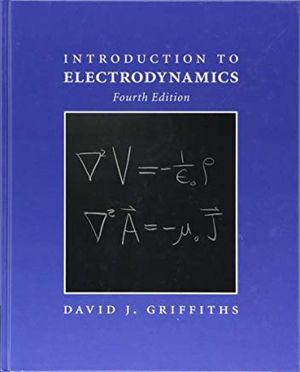Poisson's equations for electricity (top) and magnetism (bottom) in SI units on the front cover of an undergraduate textbook.

Poisson's well-known generalization of Laplace's second order partial differential equation for potential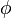$\phi$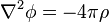$\nabla^2 \phi = - 4 \pi \rho \;$

is known as Poisson's equation after him, was first published in the Bulletin de la société philomatique (1813). If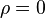$\rho = 0$, we retrieve Laplace's equation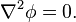$\nabla^2 \phi = 0. \;$

If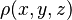$\rho(x, y, z)$ is a continuous function and if for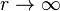$r \rightarrow \infty$ (or if a point 'moves' to infinity) a function$\phi$ goes to 0 fast enough, a solution of Poisson's equation is the Newtonian potential of a function$\rho (x, y, z)$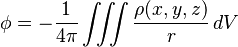$\phi = - {1\over 4 \pi} \iiint \frac{\rho (x, y, z)}{ r} \, dV \;$

where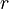$r$ is a distance between a volume element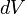$dV$and a point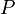$P$. The integration runs over the whole space.

Poisson's two most important memoirs on the subject are Sur l'attraction des sphéroides (Connaiss. ft. temps, 1829), and Sur l'attraction d'un ellipsoide homogène (Mim. ft. l'acad., 1835).

Poisson discovered that Laplace's equation is valid only outside of a solid. A rigorous proof for masses with variable density was first given by Carl Friedrich Gauss in 1839. Poisson's equation is applicable in not just gravitation, but also electricity and magnetism.

#### Electricity and magnetism

As the eighteenth century came to a close, human understanding of electrostatics approached maturity. Benjamin Franklin had already established the notion of electric charge and the conservation of charge; Charles-Augustin de Coulomb had enunciated his inverse-square law of electrostatics. In 1777, Joseph-Louis Lagrange introduced the concept of a potential function that can be used to compute the gravitational force of an extended body. In 1812, Poisson adopted this idea and obtained the appropriate expression for electricity, which relates the potential function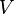$V$ to the electric charge density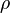$\rho$. Poisson's work on potential theory inspired George Green's 1828 paper, An Essay on the Application of Mathematical Analysis to the Theories of Electricity and Magnetism.

In 1820, Hans Christian Ørsted demonstrated that it was possible to deflect a magnetic needle by closing or opening an electric circuit nearby, resulting in a deluge of published papers attempting to explain the phenomenon. Ampère's law and the Biot-Savart law were quickly deduced. The science of electromagnetism was born. Poisson was also investigating the phenomenon of magnetism at this time, though he insisted on treating electricity and magnetism as separate phenomena. He published two memoirs on magnetism in 1826. By the 1830s, a major research question in the study of electricity was whether or not electricity was a fluid or fluids distinct from matter, or something that simply acts on matter like gravity. Coulomb, Ampère, and Poisson thought that electricity was a fluid distinct from matter. In his experimental research, starting with electrolysis, Michael Faraday sought to show this was not the case. Electricity, Faraday believed, was a part of matter.

### Optics

Poisson was a member of the academic "old guard" at the Académie royale des sciences de l'Institut de France, who were staunch believers in the particle theory of light and were skeptical of its alternative, the wave theory. In 1818, the Académie set the topic of their prize as diffraction. One of the participants, civil engineer and opticist Augustin-Jean Fresnel submitted a thesis explaining diffraction derived from analysis of both the Huygens–Fresnel principle and Young's double slit experiment.

Poisson studied Fresnel's theory in detail and looked for a way to prove it wrong. Poisson thought that he had found a flaw when he demonstrated that Fresnel's theory predicts an on-axis bright spot in the shadow of a circular obstacle blocking a point source of light, where the particle-theory of light predicts complete darkness. Poisson argued this was absurd and Fresnel's model was wrong. (Such a spot is not easily observed in everyday situations, because most everyday sources of light are not good point sources.)

The head of the committee, Dominique-François-Jean Arago, performed the experiment. He molded a 2 mm metallic disk to a glass plate with wax. To everyone's surprise he observed the predicted bright spot, which vindicated the wave model. Fresnel won the competition.

After that, the corpuscular theory of light was dead, but was revived in the twentieth century in a different form, wave-particle duality. Arago later noted that the diffraction bright spot (which later became known as both the Arago spot and the Poisson spot) had already been observed by Joseph-Nicolas Delisle and Giacomo F. Maraldi a century earlier.

### Pure mathematics and statistics

In pure mathematics, Poisson's most important works were his series of memoirs on definite integrals and his discussion of Fourier series, the latter paving the way for the classic researches of Peter Gustav Lejeune Dirichlet and Bernhard Riemann on the same subject; these are to be found in the Journal of the École Polytechnique from 1813 to 1823, and in the Memoirs de l'Académie for 1823. He also studied Fourier integrals.

Poisson wrote an essay on the calculus of variations (Mem. de l'acad., 1833), and memoirs on the probability of the mean results of observations (Connaiss. d. temps, 1827, &c). The Poisson distribution in probability theory is named after him.

In 1820 Poisson studied integrations along paths in the complex plane, becoming the first person to do so.

In 1829, Poisson published a paper on elastic bodies that contained a statement and proof of a special case of what became known as the divergence theorem.

### Mechanics

#### Analytical mechanics and the calculus of variations

Founded mainly by Leonhard Euler and Joseph-Louis Lagrange in the eighteenth century, the calculus of variations saw further development and applications in the nineteenth.

Letwhere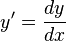$y' = \frac{dy}{dx}$. Then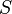$S$ is extremized if it satisfies the Euler–Lagrange equationsBut if$S$ depends on higher-order derivatives of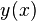$y(x)$, that is, if then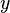$y$ must satisfy the Euler–Poisson equation,Poisson's Traité de mécanique (2 vols. 8vo, 1811 and 1833) was written in the style of Laplace and Lagrange and was long a standard work.

Let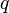$q$ be the position,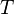$T$ be the kinetic energy,$V$ the potential energy, both independent of time$t$. Lagrange's equation of motion readsHere, the dot notation for the time derivative is used,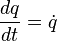$\frac{dq}{dt} = \dot{q}$. Poisson set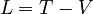$L = T - V$. He argued that if$V$ is independent of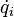$\dot{q}_i$, he could writegiving He introduced an explicit formula for momenta,Thus, from the equation of motion, he gotPoisson's text influenced the work of William Rowan Hamilton and Carl Gustav Jacob Jacobi. A translation of Poisson's Treatise on Mechanics was published in London in 1842. Let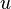$u$ and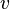$v$ be functions of the canonical variables of motion$q$ and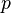$p$. Then their Poisson bracket is given byEvidently, the operation anti-commutes. More precisely,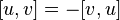$[u, v] = -[v, u]$. By Hamilton's equations of motion, the total time derivative of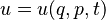$u = u (q, p, t)$ iswhere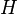$H$ is the Hamiltonian. In terms of Poisson brackets, then, Hamilton's equations can be written as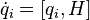$\dot{q}_i = [q_i, H]$ and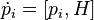$\dot{p}_i = [p_i, H]$.

Suppose$u$ is a constant of motion, then it must satisfyMoreover, Poisson's theorem states the Poisson bracket of any two constants of motion is also a constant of motion.

In September 1925, Paul Dirac received proofs of a seminal paper by Werner Heisenberg on the new branch of physics known as quantum mechanics. Soon he realized that the key idea in Heisenberg's paper was the anti-commutativity of dynamical variables and remembered that the analogous mathematical construction in classical mechanics was Poisson brackets. He found the treatment he needed in E. T. Whittaker's Analytical Dynamics of Particles and Rigid Bodies.

#### Continuum mechanics and fluid flow

In 1821, using an analogy with elastic bodies, Claude-Louis Navier arrived at the basic equations of motion for viscous fluids, now identified as the Navier–Stokes equations. In 1829 Poisson independently obtained the same result. George Gabriel Stokes re-derived them in 1845 using continuum mechanics. Poisson, Augustin-Louis Cauchy, and Sophie Germain were the main contributors to the theory of elasticity in the nineteenth century. The calculus of variations was frequently used to solve problems.

#### Wave propagation

Poisson also published a memoir on the theory of waves (Mém. ft. l'acad., 1825).

### Thermodynamics

In his work on heat conduction, Joseph Fourier maintained that the arbitrary function may be represented as an infinite trigonometric series and made explicit the possibility of expanding functions in terms of Bessel functions and Legendre polynomials, depending on the context of the problem. It took some time for his ideas to be accepted as his use of mathematics was less than rigorous. Although initially skeptical, Poisson adopted Fourier's method. From around 1815 he studied various problems in heat conduction. He published his Théorie mathématique de la chaleur in 1835.

During the early 1800s, Pierre-Simon de Laplace developed a sophisticated, if speculative, description of gases based on the old caloric theory of heat, to which younger scientists such as Poisson were less committed. A success for Laplace was his correction of Newton's formula for the speed of sound in air that gives satisfactory answers when compared with experiments. The Newton–Laplace formula makes use of the specific heats of gases at constant volume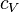$c_V$and at constant pressure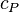$c_P$. In 1823 Poisson redid his teacher's work and reached the same results without resorting to complex hypotheses previously employed by Laplace. In addition, by using the gas laws of Robert Boyle and Joseph Louis Gay-Lussac, Poisson obtained the equation for gases undergoing adiabatic changes, namely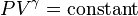$PV^{\gamma} = \text{constant}$, where$P$ is the pressure of the gas,$V$ its volume, and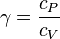$\gamma = \frac{c_P}{c_V}$.

### Other works

Besides his many memoirs, Poisson published a number of treatises, most of which were intended to form part of a great work on mathematical physics, which he did not live to complete. Among these may be mentioned:

• Nouvelle théorie de l'action capillaire (4to, 1831);
• Recherches sur la probabilité des jugements en matières criminelles et matière civile (4to, 1837), all published at Paris.
• A catalog of all of Poisson's papers and works can be found in Oeuvres complétes de François Arago, Vol. 2
• Mémoire sur l'équilibre et le mouvement des corps élastiques (v. 8 in Mémoires de l'Académie Royale des Sciences de l'Institut de France, 1829), digitized copy from the Bibliothèque nationale de France
• Recherches sur le Mouvement des Projectiles dans l'Air, en ayant égard a leur figure et leur rotation, et a l'influence du mouvement diurne de la terre (1839)

## Interaction with Évariste Galois

After political activist Évariste Galois had returned to mathematics after his expulsion from the École Normale, Poisson asked him to submit his work on the theory of equations, which he did January 1831. In early July, Poisson declared Galois' work "incomprehensible," but encouraged Galois to "publish the whole of his work in order to form a definitive opinion." While Poisson's report was made before Galois' 14 July arrest, it took until October to reach Galois in prison. It is unsurprising, in the light of his character and situation at the time, that Galois vehemently decided against publishing his papers through the academy and instead publish them privately through his friend Auguste Chevalier. Yet Galois did not ignore Poisson's advice. He began collecting all his mathematical manuscripts while still in prison, and continued polishing his ideas until his release on 29 April 1832, after which he was somehow persuaded to participate in what proved to be a fatal duel.In Spanish: Siméon Denis Poisson para niños